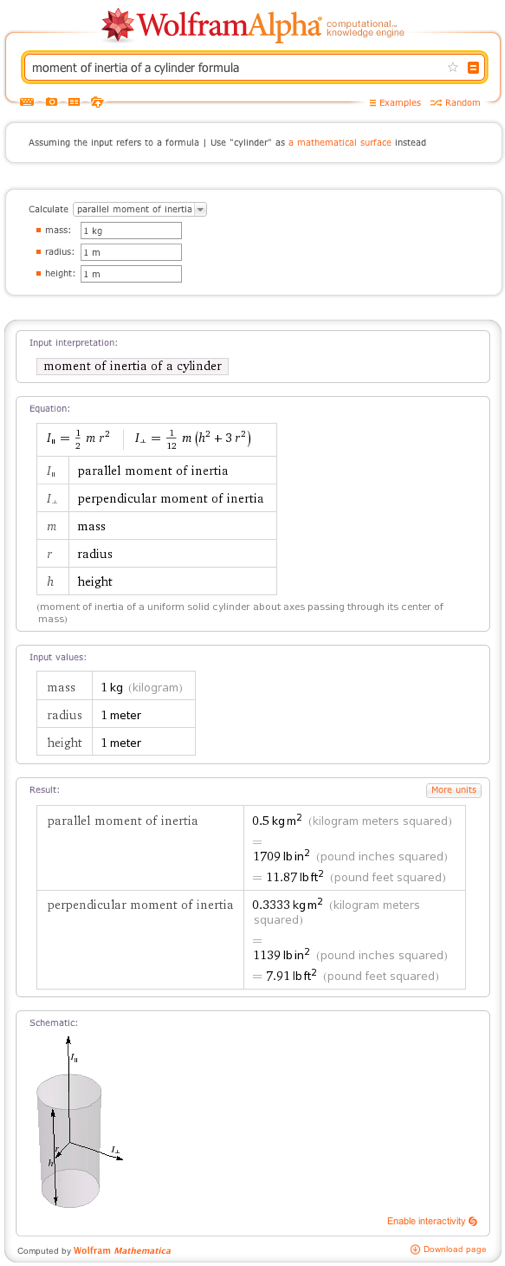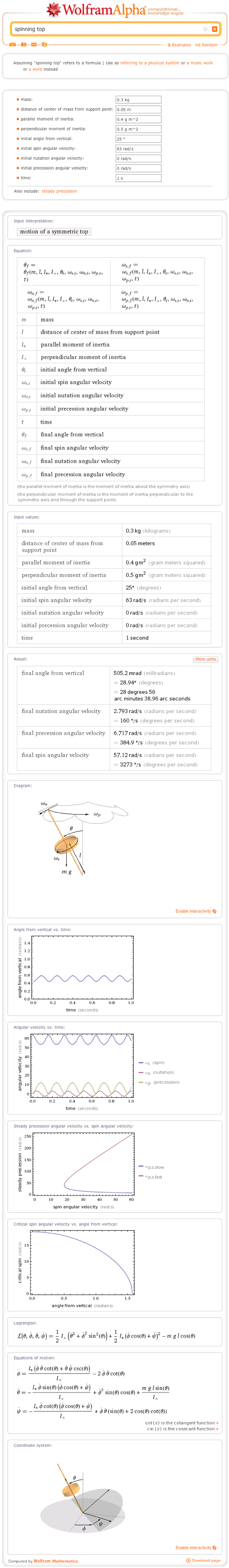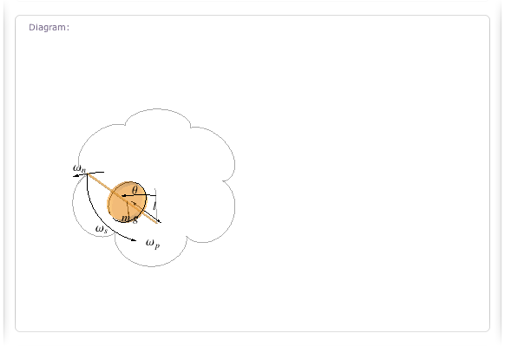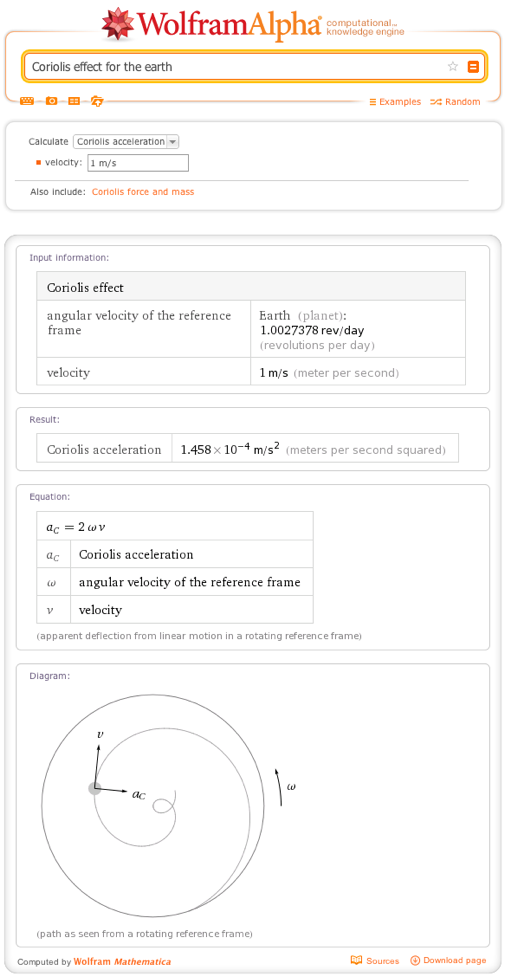# Angular Kinematics—Solving Circular Motion Problems with Wolfram|Alpha

March 20, 2013 —Comments Off

Many of us are familiar with motion in a straight line: you speed up and move faster, you travel forward and end up someplace new. But there is another type of motion: angular motion, or the motion in a circular path. These are the kinematics of a merry-go-round, a spinning top, or the orbit and rotation of the Earth.

There are many similarities between rotational motion and the more common linear motion. Most linear motion terms have a direct angular motion equivalent. For velocity we have angular velocity, the rate of change in the angular displacement of an object or the angle it makes about the circular path. Acceleration is replaced by angular acceleration, which can increase or decrease the angular velocity and is induced by torques upon the rotating object in much the same way as force induces acceleration.

Wolfram|Alpha contains many formulas for both linear and circular motion. For example, we can use the angular speed formula to find how fast a rotating object turns under a constant angular acceleration. Or we can use the angular displacement formula to find how much it has turned based on its initial angular speed and angular acceleration. Other correspondences exist. For example, in linear mechanics you have kinetic energy, the energy of a particle due to its motion. For rotating systems, you have the corresponding property of rotational kinetic energy, the energy an object possesses due to the speed of its rotation. The major difference between the two formulas is in how the inertial component is determined.

In linear motion, the mass of an object is the inertial component, and is used to determine its kinetic energy and the effect of an external force. However, in angular motion, that role is handled by the moment of inertia. The moment of inertia depends on the mass of the object as well as the distribution of that mass relative to the axis of rotation. Wolfram|Alpha contains the formulas for the moments of inertia for numerous common shapes, from rods to cubes to cylinders.We can use these tools to solve many angular motion calculations. For example, here is a simple physics word problem that a student might encounter in a first-year physics course: A wheel has a diameter of 26 inches and a width of 2 inches. It has a mass of 5 pounds and is free to rotate about its central axis. A force of 100 Newtons is applied to the edge for 5 seconds, accelerating the wheel. How fast does the wheel turn?

Using Wolfram|Alpha to solve this, we first need to determine the moment of inertia. We can approximate the wheel as a short cylinder, corresponding to the parallel moment of inertia in the previous example. Putting the mass, width, and radius (which is half of the diameter or 13 inches) into our formula, we find the moment of inertia is 422.5 pounds square inches, or 0.1236 kilograms square meters.

Placing a force along one edge of the wheel will induce a torque. A torque with a force of 100 Newtons (or roughly 22.5 pounds of force) for an object with a radius of 13 inches is 33.02 Newton meters. A torque of 33.02 Newton meters and moment of inertia of 0.1236 kilograms square meters gives us 267.15 radians per second squared. If we apply that acceleration to our wheel for 5 seconds, the angular speed formula tells us our wheel would be spinning at 1335.7 radians per second, or 212.6 revolutions per second. That’s twice the speed of a typical hard drive. To put it another way, if this wheel was rolling along the ground without slipping, it would move at 212.6 × (2 pi 13 inches/second), or 986.67 miles per hour.

For real-world calculations involving wheels of cars, you might find our tire size calculator and functionality interesting. Wolfram|Alpha can tell you what the revolutions per distance for a tire size 215/65R17 is, which you can then use to find out how far a car or other vehicle has traveled for a number of revolutions.

Another example of this kind rolling motion is a round object on an incline. The Wolfram|Alpha formula allows you to calculate the motion of a round object, like a wheel or a log, rolling down an inclined plane. You can include sliding, adjust the acceleration of gravity, and try different shapes for the object.

Another classic example of rotational motion is the spinning top. The ideal top will spin about a central axis like the wheel we discussed. But in the real world, tops have imperfections that cause their mass to be unevenly distributed, or the initial spin fails to start with the axis of rotation perfectly vertical. This causes gravity to exert a torque on its motion. This in turn creates a precession about the axis perpendicular to the main axis of rotation. Further torques can cause nutation where the path about the axis of rotation oscillates back and forth. Wolfram|Alpha includes a comprehensive formula for the motion of a top, allowing you to examine this behavior with all such factors.For those of you using Wolfram|Alpha Pro, you can use the CDF content to manipulate these diagrams to get a better look at the behavior. Here is a view from almost directly above the top:All this spinning can have profound effects on the contents of the spinning object, whether they are passengers on a roller coaster or clouds on the Earth. The primary effect felt is called centripetal acceleration, an effect many of us are familiar with when making tight turns while driving or on amusement park rides. Centripetal acceleration is the feeling you experience when traveling in a circular path. It is caused by the force that keeps you on that circular path, such as the roller coaster track pushing you inward, or your seat belt holding you in place in the car.

So how strong is this acceleration? While it clearly is fairly strong on a roller coaster, keeping people from falling out on a loop, it isn’t strong enough to throw us off the surface of the Earth. This is because it increases by the square of the rotation speed of the object and the radius of the object. But the rotational frequency of the Earth is only 1.0027378 revolutions per day. Thus the centripetal acceleration for the Earth is just 3.386 centimeters per second squared, or 0.1111 feet per second squared, which is tiny compared to the acceleration of gravity (9.8 meters per second squared).

But let’s turn this around. What would it take to simulate Earth’s gravity by using centripetal acceleration? This is a question of importance for astronauts planning to travel to other planets. Centripetal acceleration is the only alternative we currently have to a planet-sized space ship for long-term travel in space. Zero gravity conditions have a deleterious effect on the health of astronauts, particularly on the strength of their bones. If we want to walk on Mars safely when we get there, we will need some sort of artificial gravity. One way to do this is to create a rotating ring where the astronauts would live or exercise.

So how big and fast would this ring need to be? Assuming we limit the radius of our ring to 100 meters (big enough to encircle the International Space Station), the necessary angular velocity for centripetal acceleration of 9.8 m/2 at 100m would be 0.31305 radians per second, or a revolution every 20 seconds.

While the rotation of the Earth can’t counteract gravity, it can have an effect on the world’s climate through the Coriolis effect. The rotation of the Earth causes a deflection of moving objects along its surface, in the same way that the path of an ant moving forward on a record player will be twisted into a curve. These deflections are clockwise in the northern hemisphere and counterclockwise in the southern hemisphere. The effect is quite small on the Earth and is only visible over large distances or time scales, such as the motion of large storms and ocean currents.For example, the acceleration caused by the Coriolis effect for the Earth, for an object traveling at 60 miles per hour, is only 0.39 centimeters per second squared. Thus contrary to common belief, this does not commonly cause toilet and bathtub drains to spin one way in the southern hemisphere and another in the northern hemisphere. Only for a sufficiently isolated and large drain could this effect be seen, otherwise it would be overwhelmed by other influences.

A much simpler way to see the Coriolis effect is by watching a Foucault pendulum. First invented in 1851, this pendulum is allowed to swing freely in any direction. As it swings through the day, its path slowly rotates with respect to its surroundings in agreement with the Coriolis effect. This model also allows you to more easily see the cause of the Coriolis effect. In the reference frame of the pendulum, it maintains a constant path, but the Earth shifts around it, causing the seeming rotation.

Angular motion is important to many more advanced fields of physics. In cosmology, the speed of stars in a rotating galaxy was crucial in first identifying the existence of dark matter. In quantum mechanics, the quantization of angular momentum resolved the paradox of why electron orbits fail to decay. Angular motion is all around us, from the twisting of a torsion pendulum to the orbits of satellites to the rotation of molecules.

1 Comment

Great efforts and very nice animations that can hardly be outperformed

I use great animations from Wolfram in teaching physics.

As a professor I learn a lot from Wolfram animations.

Thank you very much great-minded people at Wolfram.

Posted by Mohamed Fahmy Husssein March 20, 2013 at 3:39 pm